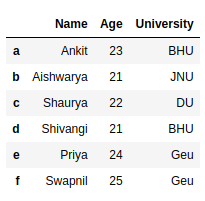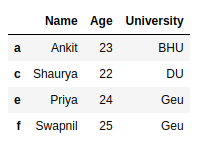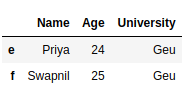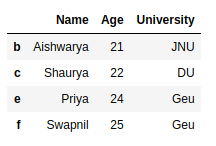GeeksforGeeks App
Open AppBrowser
Continue

# How to Drop rows in DataFrame by conditions on column values?

In this article, we are going to see several examples of how to drop rows from the dataframe based on certain conditions applied on a column.

Pandas provide data analysts a way to delete and filter data frame using `dataframe.drop()` method. We can use this method to drop such rows that do not satisfy the given conditions.

Let’s create a Pandas dataframe.

 `# import pandas library``import` `pandas as pd`` ` `# dictionary with list object in values``details ``=` `{``    ``'Name'` `: [``'Ankit'``, ``'Aishwarya'``, ``'Shaurya'``,``              ``'Shivangi'``, ``'Priya'``, ``'Swapnil'``],``    ``'Age'` `: [``23``, ``21``, ``22``, ``21``, ``24``, ``25``],``    ``'University'` `: [``'BHU'``, ``'JNU'``, ``'DU'``, ``'BHU'``, ``                    ``'Geu'``, ``'Geu'``],``}`` ` `# creating a Dataframe object ``df ``=` `pd.DataFrame(details, columns ``=` `[``'Name'``, ``'Age'``,``                                      ``'University'``],``                  ``index ``=` `[``'a'``, ``'b'``, ``'c'``, ``'d'``, ``'e'``,``                           ``'f'``])`` ` `df`

Output:Example 1 : Delete rows based on condition on a column.

 `# import pandas library``import` `pandas as pd`` ` `# dictionary with list object in values``details ``=` `{``    ``'Name'` `: [``'Ankit'``, ``'Aishwarya'``, ``'Shaurya'``,``              ``'Shivangi'``, ``'Priya'``, ``'Swapnil'``],``    ``'Age'` `: [``23``, ``21``, ``22``, ``21``, ``24``, ``25``],``    ``'University'` `: [``'BHU'``, ``'JNU'``, ``'DU'``, ``'BHU'``, ``                    ``'Geu'``, ``'Geu'``],``}`` ` `# creating a Dataframe object ``df ``=` `pd.DataFrame(details, columns ``=` `[``'Name'``, ``'Age'``,``                                      ``'University'``],``                  ``index ``=` `[``'a'``, ``'b'``, ``'c'``, ``'d'``, ``'e'``, ``'f'``])`` ` `# get names of indexes for which``# column Age has value 21``index_names ``=` `df[ df[``'Age'``] ``=``=` `21` `].index`` ` `# drop these row indexes``# from dataFrame``df.drop(index_names, inplace ``=` `True``)`` ` `df`

Output :Example 2 : Delete rows based on multiple conditions on a column.

 `# import pandas library``import` `pandas as pd`` ` `# dictionary with list object in values``details ``=` `{``    ``'Name'` `: [``'Ankit'``, ``'Aishwarya'``, ``'Shaurya'``, ``              ``'Shivangi'``, ``'Priya'``, ``'Swapnil'``],``    ``'Age'` `: [``23``, ``21``, ``22``, ``21``, ``24``, ``25``],``    ``'University'` `: [``'BHU'``, ``'JNU'``, ``'DU'``, ``'BHU'``,``                    ``'Geu'``, ``'Geu'``],``}`` ` `# creating a Dataframe object ``df ``=` `pd.DataFrame(details, columns ``=` `[``'Name'``, ``'Age'``,``                                      ``'University'``],``                  ``index ``=` `[``'a'``, ``'b'``, ``'c'``, ``'d'``, ``'e'``, ``'f'``])`` ` `# get names of indexes for which column Age has value >= 21``# and <= 23``index_names ``=` `df[ (df[``'Age'``] >``=` `21``) & (df[``'Age'``] <``=` `23``)].index`` ` `# drop these given row``# indexes from dataFrame``df.drop(index_names, inplace ``=` `True``)`` ` `df`

Output :Example 3 : Delete rows based on multiple conditions on different columns.

 `# import pandas library``import` `pandas as pd`` ` `# dictionary with list object in values``details ``=` `{``    ``'Name'` `: [``'Ankit'``, ``'Aishwarya'``, ``'Shaurya'``,``              ``'Shivangi'``, ``'Priya'``, ``'Swapnil'``],``    ``'Age'` `: [``23``, ``21``, ``22``, ``21``, ``24``, ``25``],``    ``'University'` `: [``'BHU'``, ``'JNU'``, ``'DU'``, ``'BHU'``, ``                    ``'Geu'``, ``'Geu'``],``}`` ` `# creating a Dataframe object ``df ``=` `pd.DataFrame(details, columns ``=` `[``'Name'``, ``'Age'``,``                                      ``'University'``],``                  ``index ``=` `[``'a'``, ``'b'``, ``'c'``, ``'d'``, ``'e'``, ``'f'``])`` ` `# get names of indexes for which``# column Age has value >= 21``# and column University is BHU``index_names ``=` `df[ (df[``'Age'``] >``=` `21``) & (df[``'University'``] ``=``=` `'BHU'``)].index`` ` `# drop these given row``# indexes from dataFrame``df.drop(index_names, inplace ``=` `True``)`` ` `df`

Output :My Personal Notes arrow_drop_up